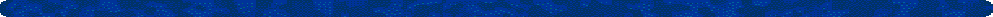Financial Ratios
P/E Ratio = Current Stock Price / 12 months of earnings
• To calculate the p/e ratio you simply divide the current price of the stock by the trailing twelve months of earnings. However, some companies and websites may use the previous calender year's earnings rather than the previous twelve months. Another variation is the forward p/e, which takes the expected earnings of the company divided by the current price. Keep in mind, the forward p/e is only an estimate as future earnings can and will fluctuate from estimates.
Current Ratio = Current Asset / Current Liabilities
• The current ratio is often used as a measure of a company's liquidity or the ability to pay off its current debt such as accounts payable and short-term notes and borrowings. The higher the number the better financial position the company is in. If the number is less than 1, then the company has more short term debt than it has "cashable" assets. This can be a red flag to potential investors as the company may have difficulty in paying future bills.
Quick Ratio = (Current Asset - Inventory)/ Current Liabilities
• The quick ratio is also a measure of a company's liquidity but it is actually a better measure than the current ratio as inventories can often take time to convert to cash. Again, the higher the number the better financial position the company is in. A quick ratio of less than 1 is still not desired, but it is not as ominous as a current ratio of less than 1.
Debt to Asset Ratio = Total Debt / Total Assets
• The debt to asset ratio is a very straight-forward ratio. The higher the ratio, the more debt the company has in respect to its assets. If the number is greater than 1, the company has more debt than it has assets. If the company has a lot of debt then it can be very susceptible to changes in the interest rate. If interest rates go up, it is likely that the monthly note payments will go up. As a result, the lower the number, or the closer the number to 0, the better financial position a company is in.
Debt to Equity Ratio = Total Debt / Shareholders Equity
• The debt to equity ratio simply measures the amount of debt a company has in respect to its outstanding common stock. Again, a company is in better financial position if the number is lower. However, with newer stock issues and/or low price stocks, be wary of companies that continue to issue more and more common stock. This dilutes the value of existing shares and the company has to earn more money just to keep its current earnings per share (EPS).
Return on Assets (ROA) = Net Income / Total Assets
• This calculation measures the amount of money a company can expect to earn on the assets it owns. For example, if the ROA is 22%, and the company has \$1,000,000 worth of assets, it can expect to earn \$220,000 in net income. This ratio is often used when a company is considering borrowing money. If they can earn a ROA acceptably higher than the prevailing loan rate, then the project should be done.
Return on Equity (ROE) = Net Income / Shareholders Equity
• This calculation measures the amount of money a company generates for its shareholders. This is one of the most popular measures of how "successful" a company is. A high ROE mean the company is successfully earning more assets and value for its shareholders off its reinvested earnings. A low ROE means that the company is not generating a good return on its reinvested earnings. The question to ask companies with a low ROE is, "If you are unable to earn a good return by reinvesting profits, then why aren't you paying out the profits in dividends?"Web www.1stock1.com
1Stock1.com
Price to Sales Ratio = Share Price / Sales Per Share
• This ratio can also be calculated by dividing the company's market cap by the revenue for the previous year. The lower the ratio, the more favorable the price/sales ratio is considered. However, because this ratio does not account for expenses it can be misleading. Some companies may have a low P/S ratio but have high expenses resulting in a low profit margin and earnings.